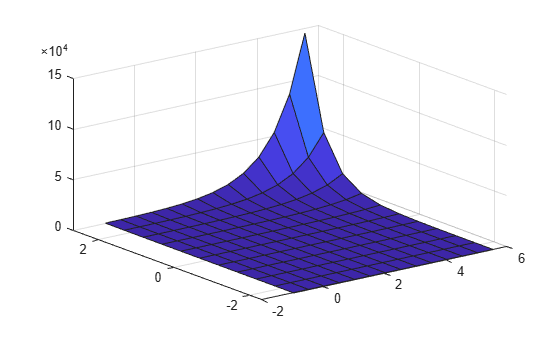Documentation

### This is machine translation

Mouseover text to see original. Click the button below to return to the English version of the page.

# feval

Evaluate generalized linear regression model prediction

## Syntax

```ypred = feval(mdl,Xnew1,Xnew2,...,Xnewn) ```

## Description

`ypred = feval(mdl,Xnew1,Xnew2,...,Xnewn)` returns the predicted response of `mdl` to the input `[Xnew1,Xnew_2,...,Xnewn]`.

## Input Arguments

 `mdl` Generalized linear model, specified as a full `GeneralizedLinearModel` object constructed using `fitglm` or `stepwiseglm`, or a compacted `CompactGeneralizedLinearModel` object constructed using `compact`. `Xnew1,Xnew2,...,Xnewn` Predictor components. `Xnewi` can be one of: ScalarVectorArray Each nonscalar component must have the same size (number of elements in each dimension). If you pass just one `Xnew` array, `Xnew` can be a table, dataset array, or an array of doubles, where each column of the array represents one predictor.

## Output Arguments

 `ypred` Predicted mean values at `Xnew`. `ypred` is the same size as each component of `Xnew`. For binomial models, `feval` uses `1` as the `BinomialSize` parameter, so `ypred` is predicted probabilities. For models with an offset, `feval` uses `0` as the offset value.

## Examples

expand all

Generate a generalized linear model, and plot its responses to a range of input data.

Generate artificial data for the model, Poisson random numbers with two underlying predictors `X(1)` and `X(2)`.

```rng('default') % for reproducibility rndvars = randn(100,2); X = [2+rndvars(:,1),rndvars(:,2)]; mu = exp(1 + X*[1;2]); y = poissrnd(mu);```

Create a generalized linear regression model of Poisson data.

`mdl = fitglm(X,y,'y ~ x1 + x2','distr','poisson');`

Generate a range of values for `X(1)` and `X(2)`, and plot the model predictions at those values.

```[Xtest1 Xtest2] = meshgrid(-1:.5:3,-2:.5:2); Z = feval(mdl,Xtest1,Xtest2); surf(Xtest1,Xtest2,Z)```## Tips

• `feval` allows you to easily evaluate predictions of a model when the model was fitted using a table or dataset array. `predict` requires a table or dataset array with the same predictor names, but you can use simple arrays of scalars with `feval`.

## Alternatives

`predict` gives the same predictions, but uses a single input array with one observation in each row, rather than one component in each input argument.

`random` predicts with added noise.